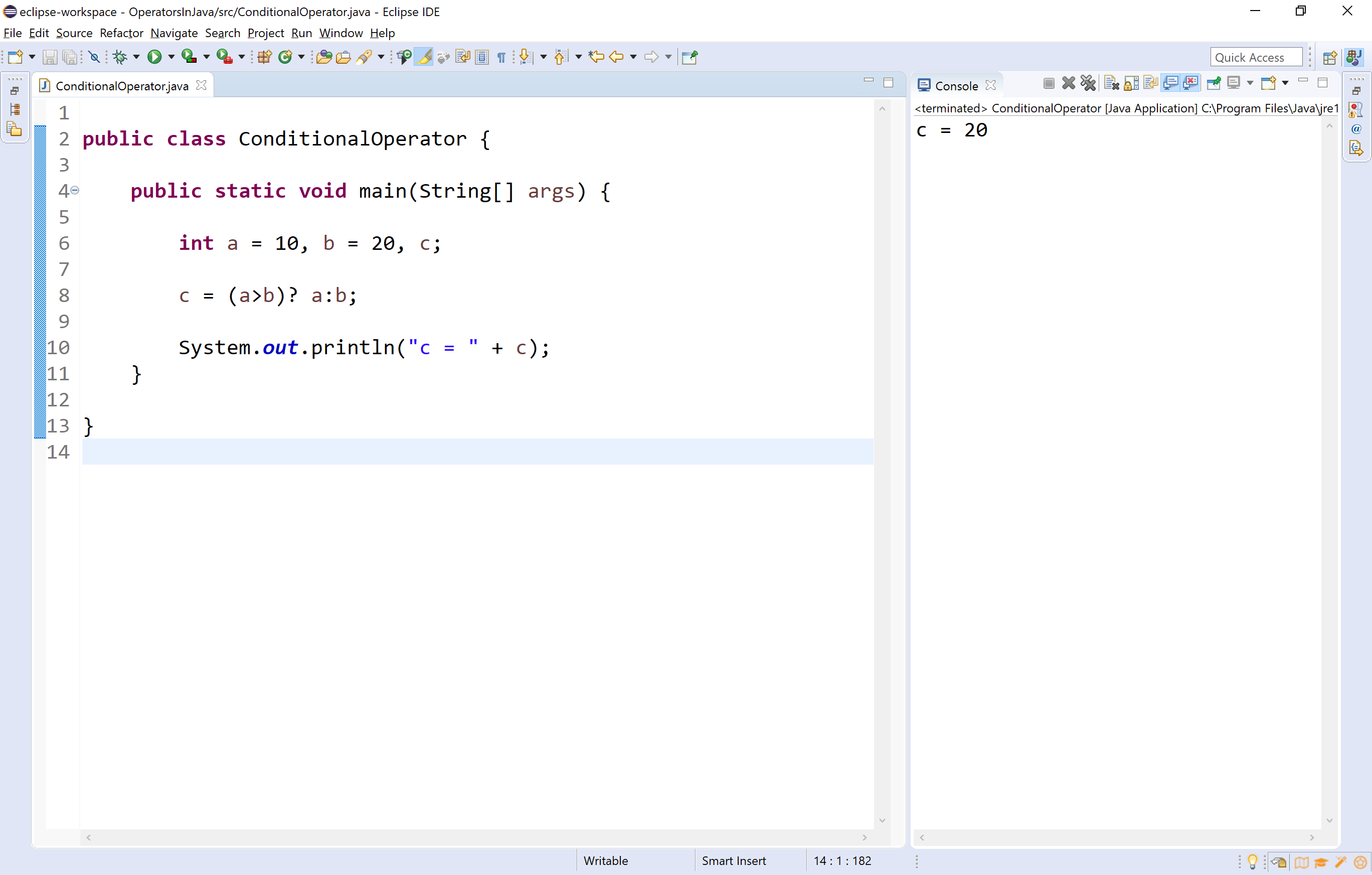The perfect place for easy learning...

×

# Java Operators

An operator is a symbol used to perform arithmetic and logical operations. Java provides a rich set of operators.

In java, operators are clasiffied into the following four types.

• Arithmetic Operqators
• Relational (or) Comparision Operators
• Logical Operators
• Assignment Operators
• Bitwise Operators
• Conditional Operators

Let's look at each operator in detail.

## Arithmetic Operators

In java, arithmetic operators are used to performing basic mathematical operations like addition, subtraction, multiplication, division, modulus, increment, decrement, etc.,

Operator Meaning Example
+ Addition 10 + 5 = 15
- Subtraction 10 - 5 = 5
* Multiplication 10 * 5 = 50
/ Division 10 / 5 = 2
% Modulus - Remainder of the Division 5 % 2 = 1
++ Increment a++
-- Decrement a--

🔔 The addition operator can be used with numerical data types and character or string data type. When it is used with numerical values, it performs mathematical addition and when it is used with character or string data type values, it performs concatination (appending).

🔔 The modulus (remainder of the division) operator is used with integer data type only.

🔔 The increment and decrement operators are used as pre-increment or pre-decrement and post-increment or post-decrement.
🔔 When they are used as pre, the value is get modified before it is used in the actual expresion and when it is used as post, the value is get modified after the the actual expression evaluation.

Let's look at the following example program.

Example
``````public class ArithmeticOperators {

public static void main(String[] args) {

int a = 10, b = 20, result;

System.out.println("a = " + a + ", b = " + b);

result = a + b;
System.out.println("Addition : " + a + " + " + b + " = " + result);

result = a - b;
System.out.println("Subtraction : " + a + " - " + b + " = " + result);

result = a * b;
System.out.println("Multiplucation : " + a + " * " + b + " = " + result);

result = b / a;
System.out.println("Division : " + b + " / " + a + " = " + result);

result = b % a;
System.out.println("Modulus : " + b + " % " + a + " = " + result);

result = ++a;
System.out.println("Pre-increment : ++a = " + result);

result = b--;
System.out.println("Post-decrement : b-- = " + result);
}
}
``````

When we run the above example code, it produces the following output.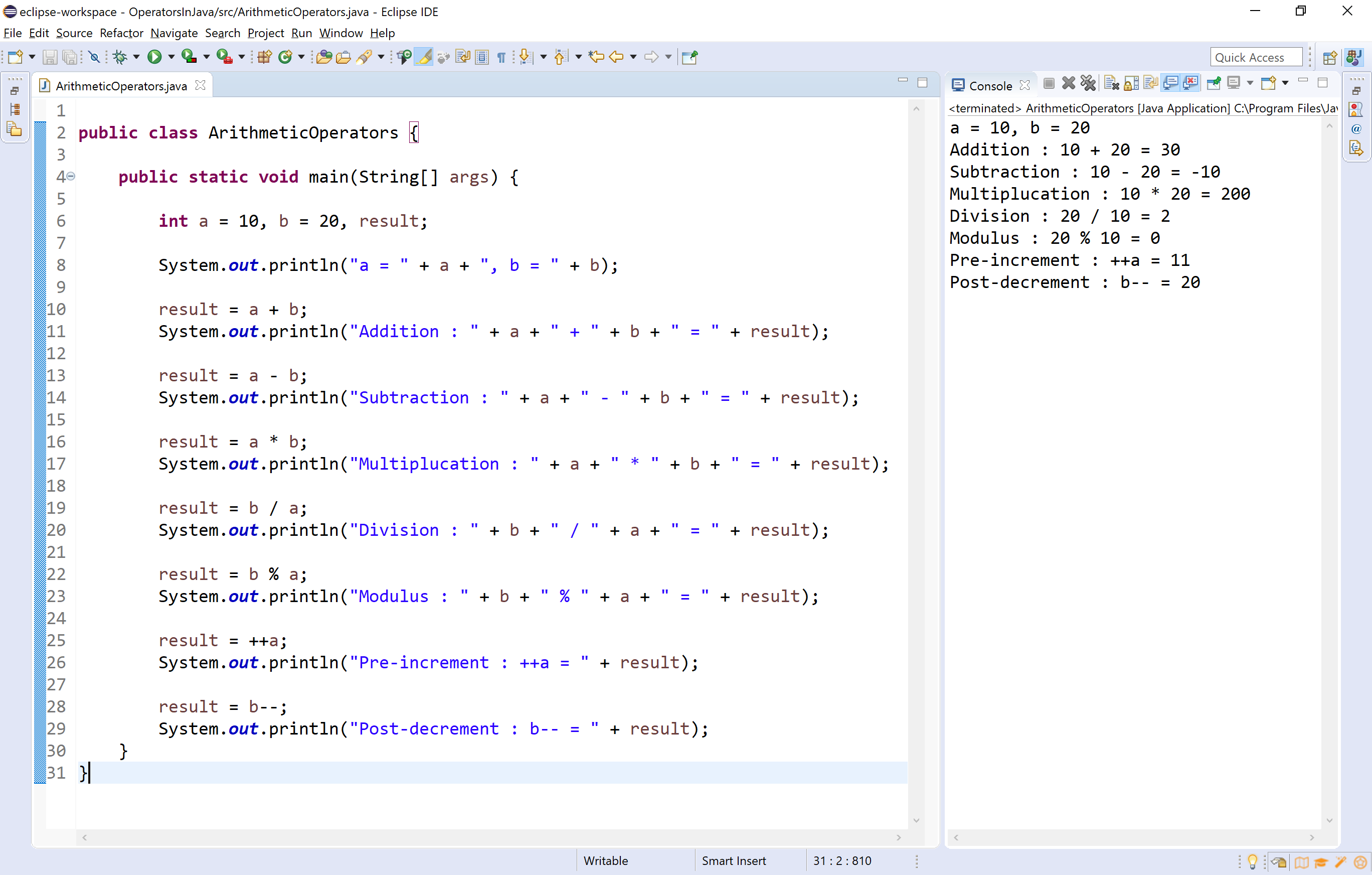## Relational Operators (<, >, <=, >=, ==, !=)

The relational operators are the symbols that are used to compare two values. That means the relational operators are used to check the relationship between two values. Every relational operator has two posible results either TRUE or FALSE. In simple words, the relational operators are used to define conditions in a program. The following table provides information about relational operators.

Operator Meaning Example
< Returns TRUE if the first value is smaller than second value otherwise returns FALSE 10 < 5 is FALSE
> Returns TRUE if the first value is larger than second value otherwise returns FALSE 10 > 5 is TRUE
<= Returns TRUE if the first value is smaller than or equal to second value otherwise returns FALSE 10 <= 5 is FALSE
>= Returns TRUE if the first value is larger than or equal to second value otherwise returns FALSE 10 >= 5 is TRUE
== Returns TRUE if both values are equal otherwise returns FALSE 10 == 5 is FALSE
!= Returns TRUE if both values are not equal otherwise returns FALSE 10 != 5 is TRUE

Look at the following example program.

Example
``````public class RelationalOperators {

public static void main(String[] args) {

boolean a;

a = 10<5;
System.out.println("10 < 5 is " + a);

a = 10>5;
System.out.println("10 > 5 is " + a);

a = 10<=5;
System.out.println("10 <= 5 is " + a);

a = 10>=5;
System.out.println("10 >= 5 is " + a);

a = 10==5;
System.out.println("10 == 5 is " + a);

a = 10!=5;
System.out.println("10 != 5 is " + a);
}

}
``````

When we run the above example code, it produces the following output.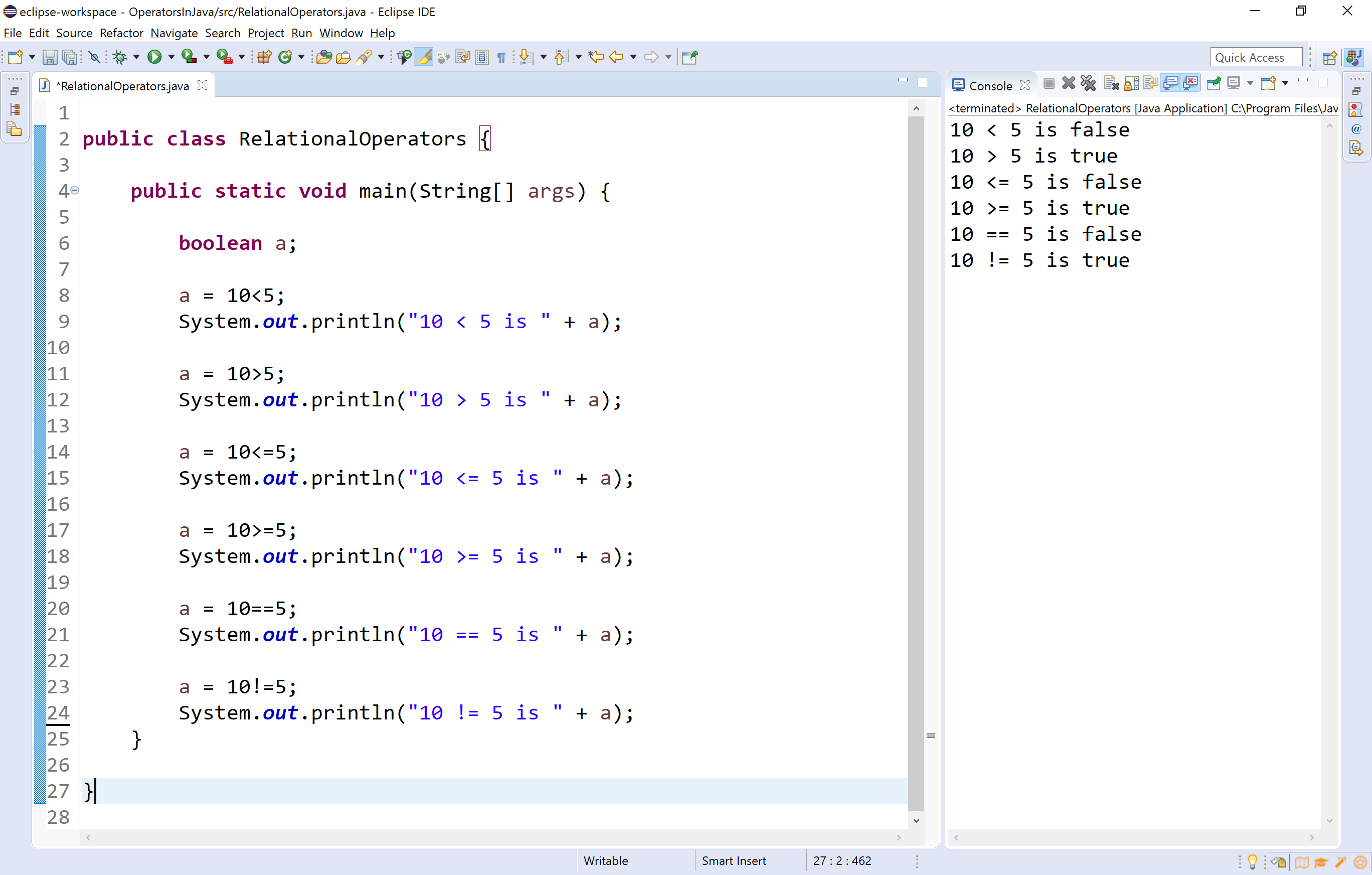## Logical Operators

The logical operators are the symbols that are used to combine multiple conditions into one condition. The following table provides information about logical operators.

Operator Meaning Example
& Logical AND - Returns TRUE if all conditions are TRUE otherwise returns FALSE false & true => false
| Logical OR - Returns FALSE if all conditions are FALSE otherwise returns TRUE false | true => true
^ Logical XOR - Returns FALSE if all conditions are same otherwise returns TRUE true ^ true => false
! Logical NOT - Returns TRUE if condition is FLASE and returns FALSE if it is TRUE !false => true
&& short-circuit AND - Similar to Logical AND (&), but once a decision is finalized it does not evaluate remianing. false & true => false
|| short-circuit OR - Similar to Logical OR (|), but once a decision is finalized it does not evaluate remianing. false | true => true

🔔 The operators &, |, and ^ can be used with both boolean and integer data type values. When they are used with integers, performs bitwise operations and with boolean, performs logical operations.

🔔 Logical operators and Short-circuit operators both are similar, but in case of short-circuit operators once the decision is finalized it does not evaluate remaining expressions.

Look at the following example program.

Example
``````public class LogicalOperators {

public static void main(String[] args) {

int x = 10, y = 20, z = 0;
boolean a = true;

a = x>y && (z=x+y)>15;
System.out.println("a = " + a + ", and z = " + z);

a = x>y & (z=x+y)>15;
System.out.println("a = " + a + ", and z = " + z);

}

}
``````

When we run the above example code, it produces the following output.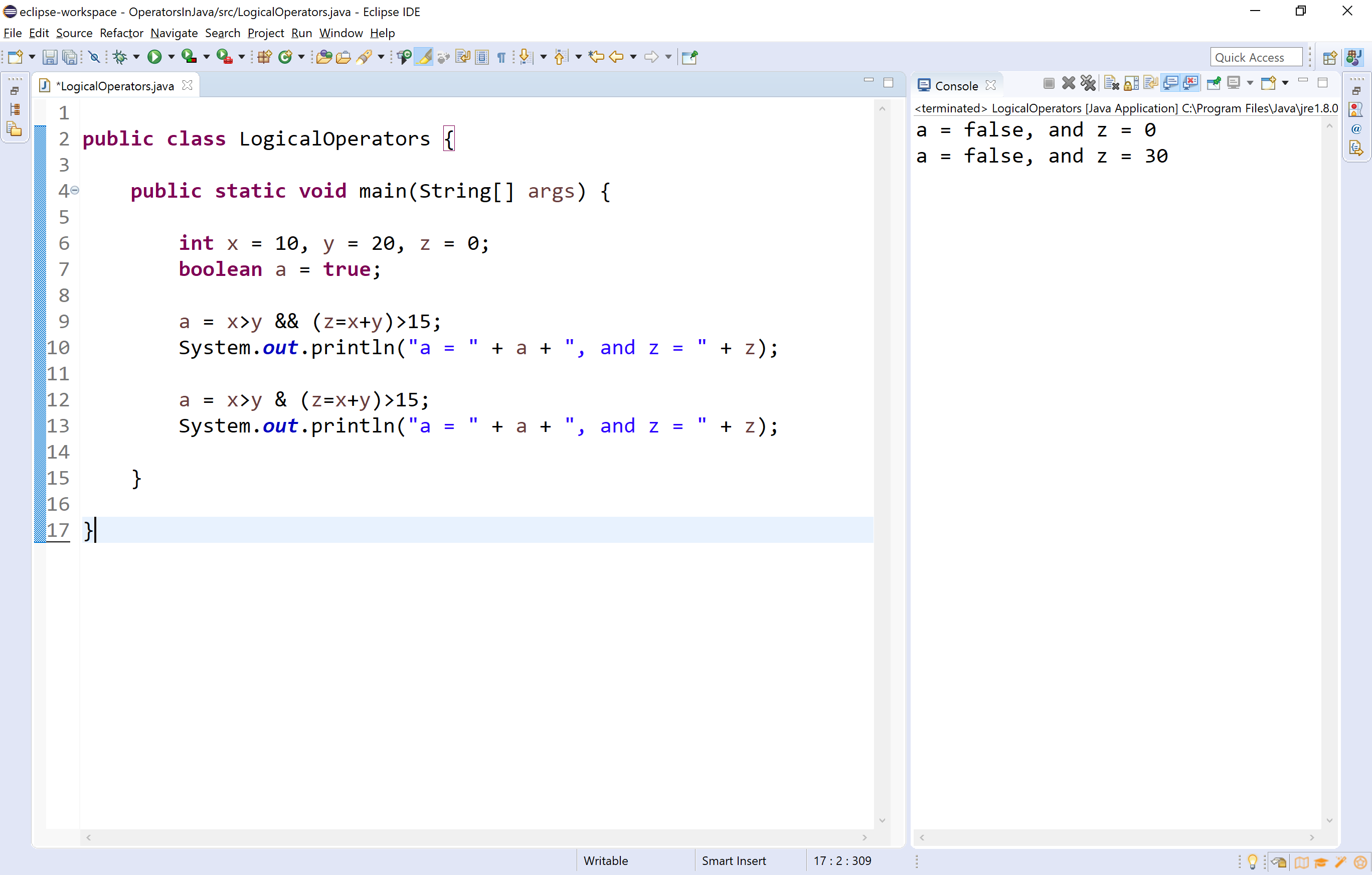## Assignment Operators

The assignment operators are used to assign right-hand side value (Rvalue) to the left-hand side variable (Lvalue). The assignment operator is used in different variants along with arithmetic operators. The following table describes all the assignment operators in the java programming language.

Operator Meaning Example
= Assign the right-hand side value to left-hand side variable A = 15
+= Add both left and right-hand side values and store the result into left-hand side variable A += 10
-= Subtract right-hand side value from left-hand side variable value and store the result into left-hand side variable A -= B
*= Multiply right-hand side value with left-hand side variable value and store the result into left-hand side variable A *= B
/= Divide left-hand side variable value with right-hand side variable value and store the result into the left-hand side variable A /= B
%= Divide left-hand side variable value with right-hand side variable value and store the remainder into the left-hand side variable A %= B
&= Logical AND assignment -
|= Logical OR assignment -
^= Logical XOR assignment -

Look at the following example program.

Example
``````public class AssignmentOperators {

public static void main(String[] args) {

int a = 10, b = 20, c;
boolean x = true;

System.out.println("a = " + a + ", b = " + b);

a += b;
System.out.println("a = " + a);

a -= b;
System.out.println("a = " + a);

a *= b;
System.out.println("a = " + a);

a /= b;
System.out.println("a = " + a);

a %= b;
System.out.println("a = " + a);

x |= (a>b);
System.out.println("x = " + x);

x &= (a>b);
System.out.println("x = " + x);
}
}
``````

When we run the above example code, it produces the following output.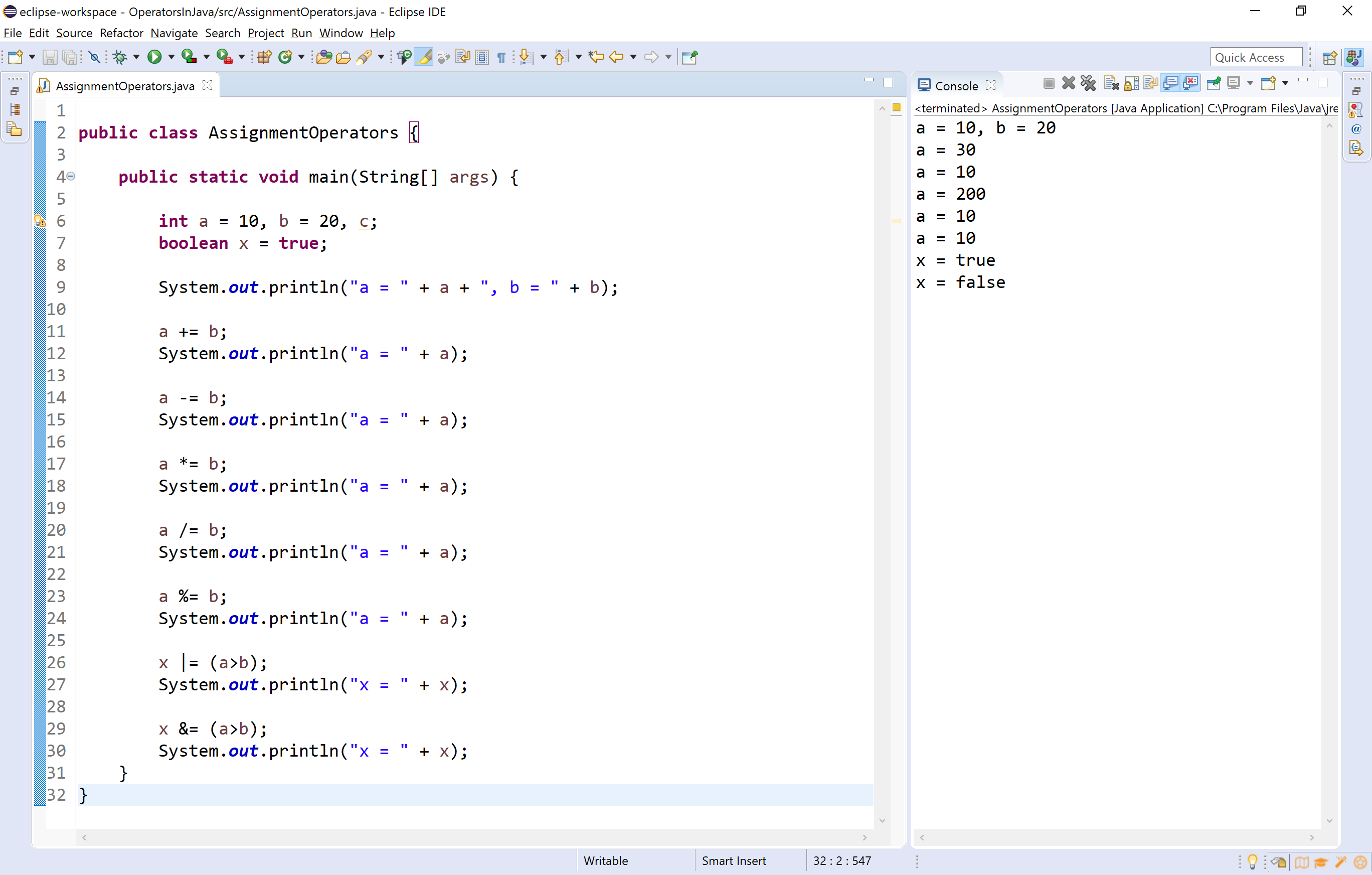## Bitwise Operators

The bitwise operators are used to perform bit-level operations in the java programming language. When we use the bitwise operators, the operations are performed based on binary values. The following table describes all the bitwise operators in the java programming language.
Let us consider two variables A and B as A = 25 (11001) and B = 20 (10100).

Operator Meaning Example
& the result of Bitwise AND is 1 if all the bits are 1 otherwise it is 0     A & B
⇒ 16 (10000)
| the result of Bitwise OR is 0 if all the bits are 0 otherwise it is 1     A | B
⇒ 29 (11101)
^ the result of Bitwise XOR is 0 if all the bits are same otherwise it is 1     A ^ B
⇒ 13 (01101)
~ the result of Bitwise once complement is negation of the bit (Flipping)     ~A
⇒ 6 (00110)
<< the Bitwise left shift operator shifts all the bits to the left by the specified number of positions     A << 2
⇒ 100 (1100100)
>> the Bitwise right shift operator shifts all the bits to the right by the specified number of positions     A >> 2
⇒ 6 (00110)

Look at the following example program.

Example
``````public class BitwiseOperators {

public static void main(String[] args) {

int a = 25, b = 20;

System.out.println(a + " & " + b + " = " + (a & b));

System.out.println(a + " | " + b + " = " + (a | b));

System.out.println(a + " ^ " + b + " = " + (a ^ b));

System.out.println("~" + a + " = " + ~a);

System.out.println(a + ">>" + 2 + " = " + (a>>2));

System.out.println(a + "<<" + 2 + " = " + (a<<2));

System.out.println(a + ">>>" + 2 + " = " + (a>>>2));
}

}
``````

When we run the above example code, it produces the following output.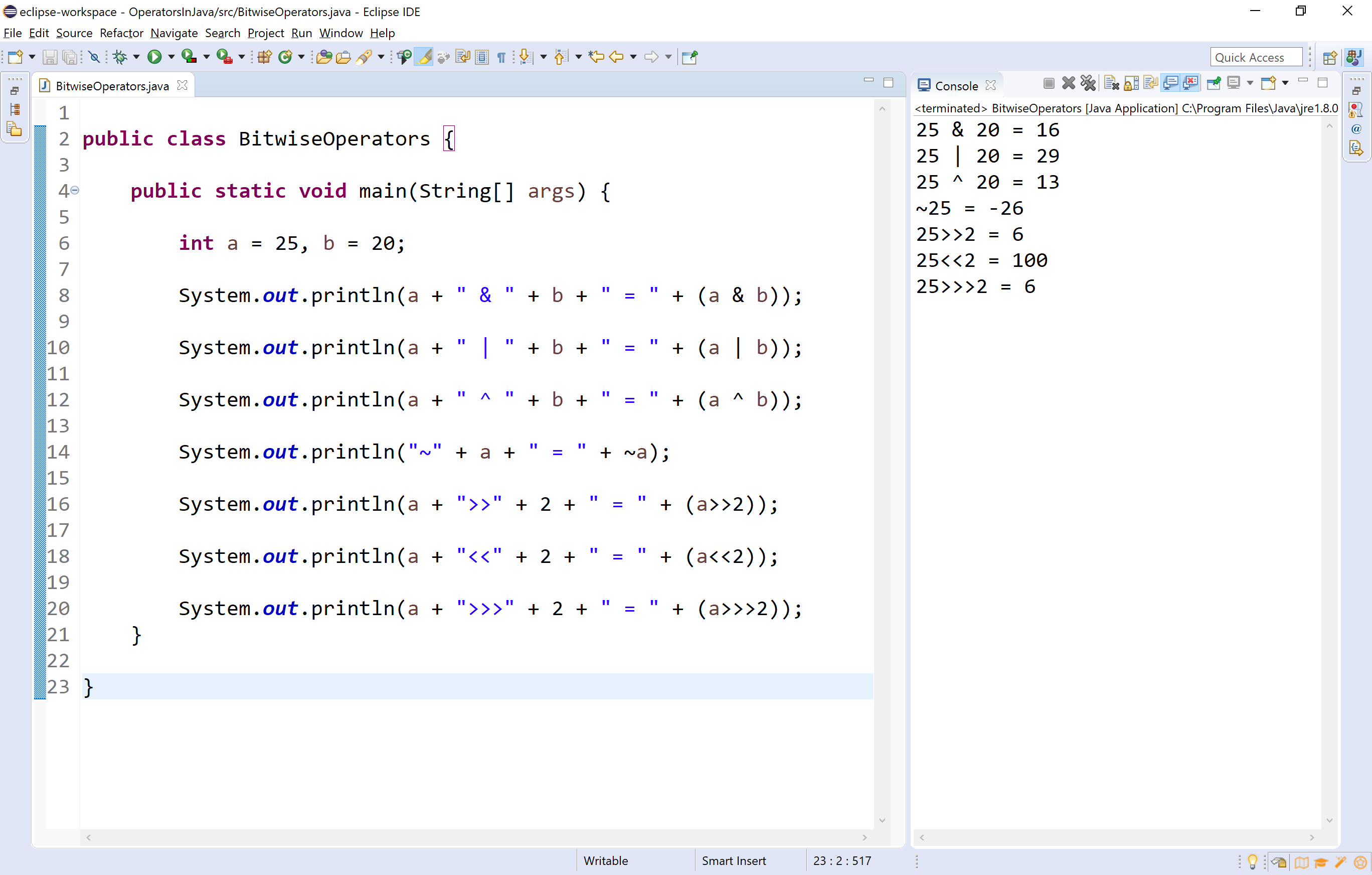## Conditional Operators

The conditional operator is also called a ternary operator because it requires three operands. This operator is used for decision making. In this operator, first, we verify a condition, then we perform one operation out of the two operations based on the condition result. If the condition is TRUE the first option is performed, if the condition is FALSE the second option is performed. The conditional operator is used with the following syntax.

Syntax
``````Condition ? TRUE Part : FALSE Part;
``````

Look at the following example program.

Example
``````public class ConditionalOperator {

public static void main(String[] args) {

int a = 10, b = 20, c;

c = (a>b)? a : b;

System.out.println("c = " + c);
}

}
``````

When we run the above example code, it produces the following output.